# Logic, Explainability and the Future of Understanding

For further developments, see wolframscience.com/metamathematics

## A Discovery about Basic Logic

Logic is a foundation for many things. But what are the foundations of logic itself?

In symbolic logic, one introduces symbols like p and q to stand for statements (or “propositions”) like “this is an interesting essay”. Then one has certain “rules of logic”, like that, for any p and any q, NOT (p AND q) is the same as (NOT pOR (NOT q).

But where do these “rules of logic” come from? Well, logic is a formal system. And, like Euclid’s geometry, it can be built on axioms. But what are the axioms? We might start with things like p AND q = q AND p, or  p = p. But how many axioms does one need? And how simple can they be?

It was a nagging question for a long time. But at 8:31pm on Saturday, January 29, 2000, out on my computer screen popped a single axiom. I had already shown there couldn’t be anything simpler, but I soon established that this one little axiom was enough to generate all of logic: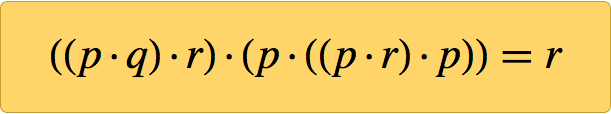✕ `((p·q)·r)·(p·((p·r)·p))==r`

But how did I know it was correct? Well, because I had a computer prove it. And here’s the proof, as I printed it in 4-point type in A New Kind of Science (and it’s now available in the Wolfram Data Repository):

With the latest version of the Wolfram Language, anyone can now generate this proof in under a minute. And given this proof, it’s straightforward to verify each step. But why is the result true? What’s the explanation?

That’s the same kind of question that’s increasingly being asked about all sorts of computational systems, and all sorts of applications of machine learning and AI. Yes, we can see what happens. But can we understand it?

I think this is ultimately a deep question—that’s actually critical to the future of science and technology, and in fact to the future of our whole intellectual development.

## The History

Logic as a formal discipline basically originated with Aristotle in the 4th century BC. As part of his lifelong effort to catalog things (animals, causes, etc.), Aristotle cataloged valid forms of arguments, and created symbolic templates for them which basically provided the main content of logic for two thousand years.

By the 1400s, however, algebra had been invented, and with it came cleaner symbolic representations of things. But it was not until 1847 that George Boole finally formulated logic in the same kind of way as algebra, with logical operations like AND and OR being thought of as operating according to algebra-like rules.

Within a few years, people were explicitly writing down axiom systems for logic. A typical example was: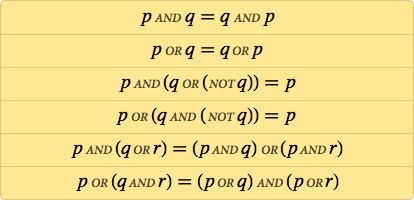✕ ```and[p,q]==and[q,p],or[p,q]==or[q,p],and[p,or[q,not[q]]]==p,or[p,and[q,not[q]]]==p,and[p,or[q,r]]==or[and[p,q],and[p,r]],or[p,and[q,r]]==and[or[p,q],or[p,r]]} ```

But does logic really need AND and OR and NOT? After the first decade of the 1900s several people had discovered that actually the single operation that we now call NAND is enough, with for example p OR q being computed as (p NAND pNAND (q NAND q). (The “functional completeness” of NAND could have remained forever a curiosity but for the development of semiconductor technology—which implements all the billions of logic operations in a modern microprocessor with combinations of transistors that perform none other than NAND or the related function NOR.)

But, OK, so what do the axioms of logic (or “Boolean algebra”) look like in terms of NAND? Here’s the first known version of them, from Henry Sheffer in 1913 (here dot · stands for NAND):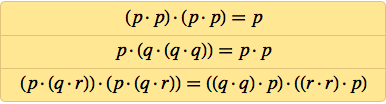✕ `{(p\[CenterDot]p)\[CenterDot](p\[CenterDot]p)==p,p\[CenterDot](q\[CenterDot](q\[CenterDot]q))==p\[CenterDot]p,(p\[CenterDot](q\[CenterDot]r))\[CenterDot](p\[CenterDot](q\[CenterDot]r))==((q\[CenterDot]q)\[CenterDot]p)\[CenterDot]((r\[CenterDot]r)\[CenterDot]p)}`

Back in 1910 Whitehead and Russell’s Principia Mathematica had popularized the idea that perhaps all of mathematics could be derived from logic. And particularly with this in mind, there was significant interest in seeing just how simple the axioms for logic could be. Some of the most notable work on this was done in Lviv and Warsaw (then both part of Poland), particularly by Jan Łukasiewicz (who, as a side effect of his work, invented in 1920 parenthesis-free Łukasiewicz or “Polish” notation). In 1944, at the age of 66, Łukasiewicz fled from the approaching Soviets—and in 1947 ended up in Ireland.

Meanwhile, the Irish-born Carew Meredith, who had been educated at Winchester and Cambridge, and had become a mathematics coach in Cambridge, had been forced by his pacifism to go back to Ireland in 1939. And in 1947, Meredith went to lectures by Łukasiewicz in Dublin, which inspired him to begin a search for simple axioms, which would occupy most of the rest of his life.

Already by 1949, Meredith found the two-axiom system: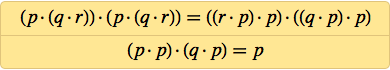✕ `{(p\[CenterDot](q\[CenterDot]r))\[CenterDot](p\[CenterDot](q\[CenterDot]r))==((r\[CenterDot]p)\[CenterDot]p)\[CenterDot]((q\[CenterDot]p)\[CenterDot]p),(p\[CenterDot]p)\[CenterDot](q\[CenterDot]p)==p}`

After nearly 20 years of work, he had succeeded by 1967 in simplifying this to:✕ `{p\[CenterDot](q\[CenterDot](p\[CenterDot]r))==((r\[CenterDot]q)\[CenterDot]q)\[CenterDot]p,(p\[CenterDot]p)\[CenterDot](q\[CenterDot]p)==p}`

But could it get any simpler? Meredith had been picking away for years trying to see how a NAND could be removed here or there. But after 1967 he apparently didn’t get any further (he died in 1976), though in 1969 he did find the three-axiom system: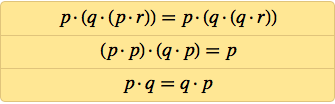✕ `{p\[CenterDot](q\[CenterDot](p\[CenterDot]r))==p\[CenterDot](q\[CenterDot](q\[CenterDot]r)),(p\[CenterDot]p)\[CenterDot](q\[CenterDot]p)==p,p\[CenterDot]q==q\[CenterDot]p}`

I actually didn’t know about Meredith’s work when I started exploring axiom systems for logic. I’d gotten into the subject as part of trying to understand what kinds of behavior simple rules could produce. Back in the early 1980s I’d made the surprising discovery that even cellular automata with some of the simplest possible rules—like my favorite rule 30—could generate behavior of great complexity.

And having spent the 1990s basically trying to figure out just how general this phenomenon was, I eventually wanted to see how it might apply to mathematics. It’s an immediate observation that in mathematics one’s basically starting from axioms (say for arithmetic, or geometry, or logic), and then trying to prove a whole collection of sophisticated theorems from them.

But just how simple can the axioms be? Well, that was what I wanted to discover in 1999. And as my first example, I decided to look at logic (or, equivalently, Boolean algebra). Contrary to what I would ever have expected beforehand, my experience with cellular automata, Turing machines, and many other kinds of systems—including even partial differential equations—was that one could just start enumerating the simplest possible cases, and after not too long one would start seeing interesting things.

But could one “discover logic” this way? Well, there was only one way to tell. And in late 1999 I set things up to start exploring what amounts to the space of all possible axiom systems—starting with the simplest ones.

In a sense any axiom system provides a set of constraints, say on p · q. It doesn’t say what p · q “is”; it just gives properties that p · q must satisfy (like, for example, it could say that p · q = q · p). Then the question is whether from these properties one can derive all the theorems of logic that hold when p · q is Nand[pq]: no more and no less.

There’s a direct way to test some of this. Just take the axiom system, and see what explicit forms of p · q satisfy the axioms if p and q can, say, be True or False. If the axiom system were just p · q = q · p then, yes, p · q could be Nand[pq]—but it doesn’t have to be. It could also be And[pq] or Equal[pq]—or lots of other things which won’t satisfy the same theorems as the NAND function in logic. But by the time one gets to the axiom system {((p · p) · q) · (q · p) = q} one’s reached the point where Nand[pq] (and the basically equivalent Nor[pq]) are the only “models” of p · q that work—at least assuming p and q have only two possible values.

So is this then an axiom system for logic? Well, no. Because it implies, for example, that there’s a possible form for p · q with 3 values for p and q, whereas there’s no such thing for logic. But, OK, the fact that this axiom system with just one axiom even gets close suggests it might be worth looking for a single axiom that reproduces logic. And that’s what I did back in January 2000 (it’s gotten a bit easier these days, thanks notably to the handy, fairly new Wolfram Language function Groupings).

It was easy to see that no axioms with 3 or fewer “NANDs” (or, really, 3 or fewer “dot operators”) could work. And by 5am on Saturday, January 29 (yes, I was a night owl then), I’d found that none with 4 NANDs could work either. By the time I stopped working on it a little after 6am, I’d gotten 14 possible candidates with 5 NANDs. But when I started work again on Saturday evening and did more tests, every one of these candidates failed.

So, needless to say, the next step was to try cases with 6 NANDs. There were 288,684 of these in all. But my code was efficient, and it didn’t take long before out popped on my screen (yes, from Mathematica Version 4):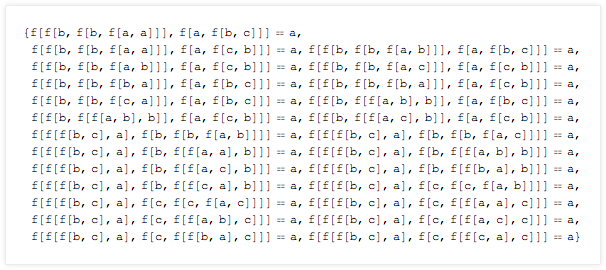At first I didn’t know what I had. All I knew was that these were the 25 inequivalent 6-NAND axioms that got further than any of the 5-NAND ones. But were any of them really an axiom system for logic? I had a (rather computation-intensive) empirical method that could rule axioms out. But the only way to know for sure whether any axiom was actually correct was to prove that it could successfully reproduce, say, the Sheffer axioms for logic.

It took a little software wrangling, but before many days had gone by, I’d discovered that most of the 25 couldn’t work. And in the end, just two survived: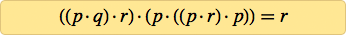✕ `{((p\[CenterDot]q)\[CenterDot]r)\[CenterDot](p\[CenterDot]((p\[CenterDot]r)\[CenterDot]p))==r}`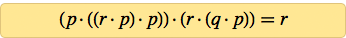✕ `{(p\[CenterDot]((r\[CenterDot]p)\[CenterDot]p))\[CenterDot](r\[CenterDot](q\[CenterDot]p))==r}`

And to my great excitement, I was successfully able to have my computer prove that both are axioms for logic. The procedure I’d used ensured that there could be no simpler axioms for logic. So I knew I’d come to the end of the road: after a century (or maybe even a couple of millennia), we could finally say that the simplest possible axiom for logic was known.

Not long after, I found two 2-axiom systems, also with 6 NANDs in total, that I proved could reproduce logic: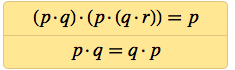✕ `{(p\[CenterDot]q)\[CenterDot](p\[CenterDot](q\[CenterDot]r))==p,p\[CenterDot]q==q\[CenterDot]p}`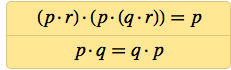✕ `{(p\[CenterDot]r)\[CenterDot](p\[CenterDot](q\[CenterDot]r))==p,p\[CenterDot]q==q\[CenterDot]p}`

And if one chooses to take commutativity p · q = q · p for granted, then these show that all it takes to get logic is one tiny 4-NAND axiom.

## Why It Matters

OK, so it’s neat to be able to say that one’s “finished what Aristotle started” (or at least what Boole started) and found the very simplest possible axiom system for logic. But is it just a curiosity, or is there real significance to it?

Before the whole framework I developed in A New Kind of Science, I think one would have been hard-pressed to view it as much more than a curiosity. But now one can see that it’s actually tied into all sorts of foundational questions, like whether one should consider mathematics to be invented or discovered.

Mathematics as humans practice it is based on a handful of particular axiom systems—each in effect defining a certain field of mathematics (say logic, or group theory, or geometry, or set theory). But in the abstract, there are an infinite number of possible axiom systems out there—in effect each defining a field of mathematics that could in principle be studied, even if we humans haven’t ever done it.

Before A New Kind of Science I think I implicitly assumed that pretty much anything that’s just “out there” in the computational universe must somehow be “less interesting” than things we humans have explicitly built and studied. But my discoveries about simple programs made it clear that at the very least there’s often lots of richness in systems that are just “out there” than in ones that we carefully select.

So what about axiom systems for mathematics? Well, to compare what’s just “out there” with what we humans have studied, we have to know where the axiom systems for existing areas of mathematics that we’ve studied—like logic—actually lie. And based on traditional human-constructed axiom systems we’d conclude that they have to be far, far out there—in effect only findable if one already knows where they are.

But my axiom-system discovery basically answered the question, “How far out is logic?” For something like cellular automata, it’s particularly easy to assign a number (as I did in the early 1980s) to each possible cellular automaton. It’s slightly harder to do this with axiom systems, though not much. And in one approach, my axiom can be labeled as 411;3;7;118—constructed in the Wolfram Language as: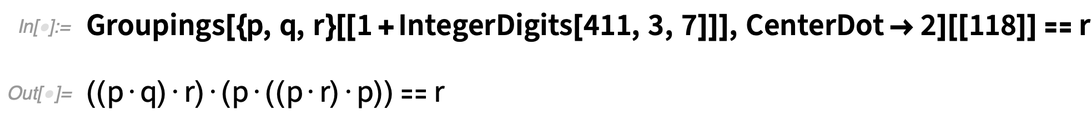✕ `Groupings[{p, q, r}[[1 + IntegerDigits[411, 3, 7]]], CenterDot -> 2][] == r`

And at least in the space of possible functional forms (not accounting for variable labeling), here’s a visual indication of where the axiom lies:Given how fundamental logic is to so many formal systems we humans study, we might have thought that in any reasonable representation, logic corresponds to one of the very simplest conceivable axiom systems. But at least with the (NAND-based) representation we’re using, that’s not true. There’s still by most measures a very simple axiom system for it, but it’s perhaps the hundred thousandth possible axiom system one would encounter if one just started enumerating axiom systems starting from the simplest one.

So given this, the obvious next question is, what about all the other axiom systems? What’s the story with those? Well, that’s exactly the kind of investigation that A New Kind of Science is all about. And indeed in the book I argue that things like the systems we see in nature are often best captured precisely by those “other rules” that we can find by enumerating possibilities.

In the case of axiom systems, I made a picture that represents what happens in “fields of mathematics” corresponding to different possible axiom systems. Each row shows the consequences of a particular axiom system, with the boxes across the page indicating whether a particular theorem is true in that axiom system. (Yes, at some point Gödel’s Theorem bites one, and it becomes irreducibly difficult to prove or disprove a given theorem in a given axiom system; in practice, with my methods that happened just a little further to the right than the picture shows…)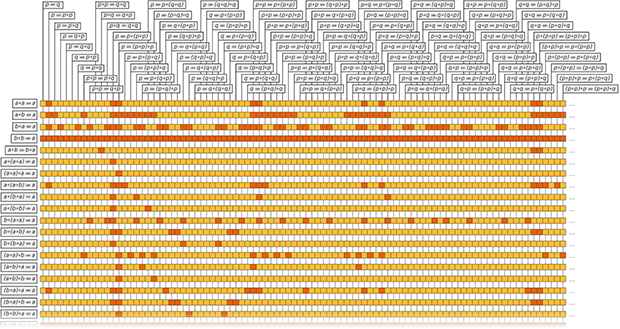Is there something fundamentally special about “human-investigated” fields of mathematics? From this picture, and other things I’ve studied, there doesn’t seem to be anything obvious. And I suspect actually that the only thing that’s really special about these fields of mathematics is the historical fact that they are what have been studied. (One might make claims like that they arise because they “describe the real world”, or because they’re “related to how our brains work”, but the results in A New Kind of Science argue against these.)

Alright, well then what’s the significance of my axiom system for logic? The size of it gives a sense of the ultimate information content of logic as an axiomatic system. And it makes it look like—at least for now—we should view logic as much more having been “invented as a human construct” than having been “discovered” because it was somehow “naturally exposed”.

If history had been different, and we’d routinely looked (in the manner of A New Kind of Science) at lots of possible simple axiom systems, then perhaps we would have “discovered” the axiom system for logic as one with particular properties we happened to find interesting. But given that we have explored so few of the possible simple axiom systems, I think we can only reasonably view logic as something “invented”—by being constructed in an essentially “discretionary” way.

In a sense this is how logic looked, say, back in the Middle Ages—when the possible syllogisms (or valid forms of argument) were represented by (Latin) mnemonics like bArbArA and cElErAnt. And to mirror this, it’s fun to find mnemonics for what we now know is the simplest possible axiom system for logic.

Starting with ((p · q) · r) · (p · ((p · r) · p)) = r, we can represent each p · q in prefix or Polish form (the reverse of the “reverse Polish” of an HP calculator) as Dpq—so the whole axiom can be written =DDDpqrDpDDprpr. Then (as Ed Pegg found for me) there’s an English mnemonic for this: FIGure OuT Queue, where p, q, r are u, r, e. Or, looking at first letters of words (with operator B, and p, q, r being a, p, c): “Bit by bit, a program computed Boolean algebra’s best binary axiom covering all cases”.

## The Mechanics of Proof

OK, so how does one actually prove that my axiom system is correct? Well, the most immediate thing to do is just to show that from it one can derive a known axiom system for logic—like Sheffer’s axiom system: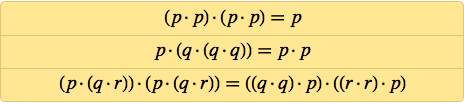✕ `{(p\[CenterDot]p)\[CenterDot](p\[CenterDot]p)==p,p\[CenterDot](q\[CenterDot](q\[CenterDot]q))==p\[CenterDot]p,(p\[CenterDot](q\[CenterDot]r))\[CenterDot](p\[CenterDot](q\[CenterDot]r))==((q\[CenterDot]q)\[CenterDot]p)\[CenterDot]((r\[CenterDot]r)\[CenterDot]p)}`

There are three axioms here, and we’ve got to derive each of them. Well, with the latest version of the Wolfram Language, here’s what we do to derive, say, the second axiom: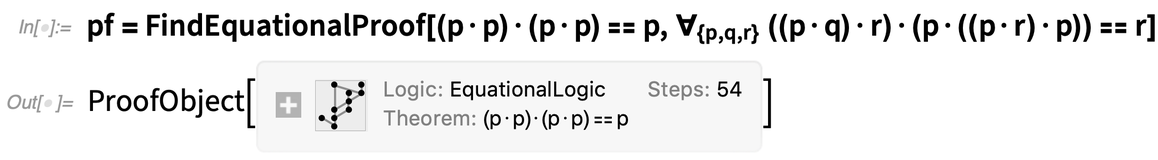✕ ```pf = FindEquationalProof[ p\[CenterDot](q\[CenterDot](q\[CenterDot]q)) == p\[CenterDot]p, \!\( \*SubscriptBox[\(\[ForAll]\), \({p, q, r}\)]\(\((\((p\[CenterDot]q)\)\[CenterDot]r)\)\[CenterDot]\((p\ \[CenterDot]\((\((p\[CenterDot]r)\)\[CenterDot]p)\))\) == r\)\)]```

It’s pretty remarkable that it’s now possible to just do this. The “proof object” records that 125 steps were used in the proof. And from this proof object we can generate a notebook that describes each of those steps: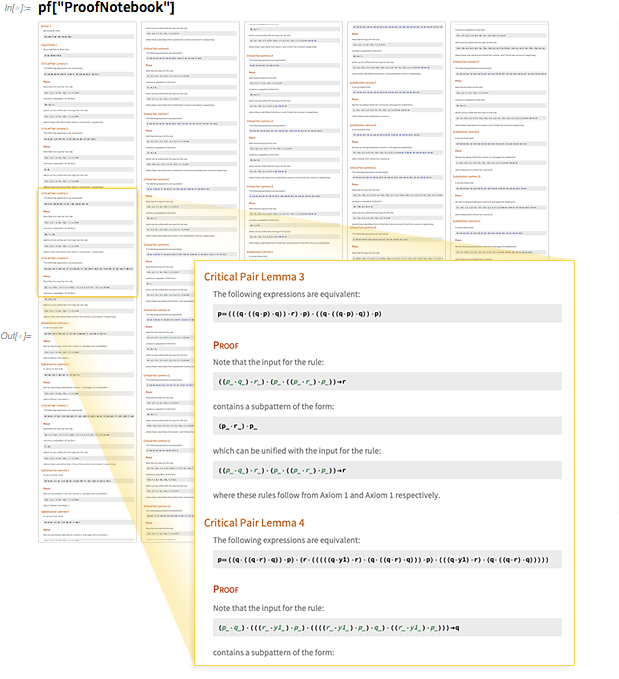✕ `pf["ProofNotebook"]`

In outline, what happens is that a whole sequence of intermediate lemmas are proved, which eventually allow the final result to be derived. There’s a whole network of interdependencies between lemmas, as this visualization shows: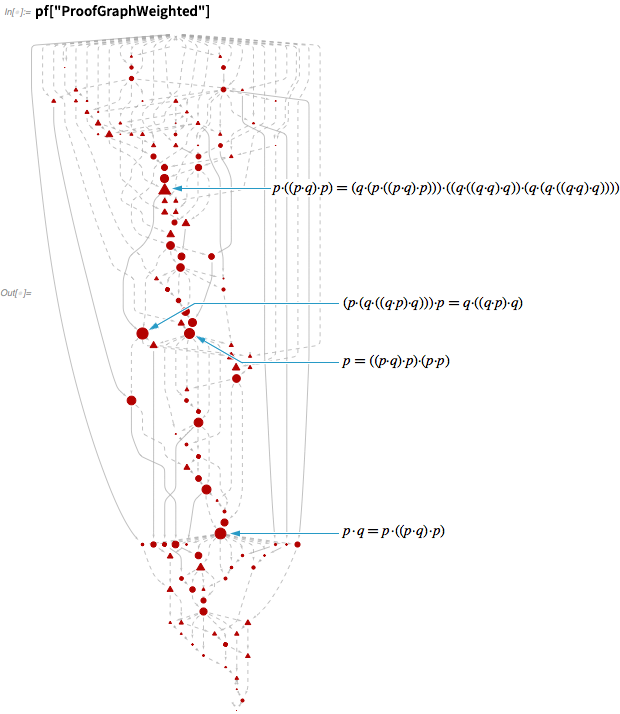✕ `pf["ProofGraphWeighted"]`

Here are the networks involved in deriving all three of the axioms in the Sheffer axiom system—with the last one involving a somewhat whopping 504 steps: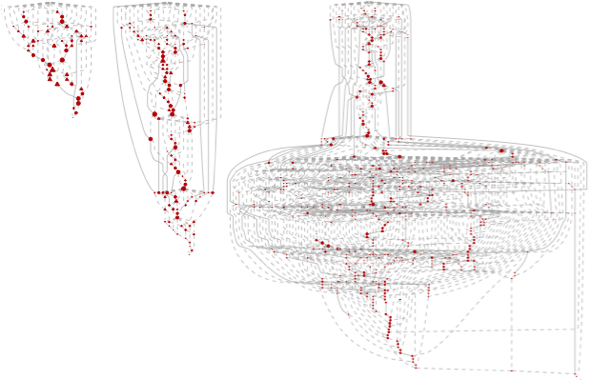And, yes, it’s clear these are pretty complicated. But before we discuss what that complexity means, let’s talk about what actually goes on in the individual steps of these proofs.

The basic idea is straightforward. Let’s imagine we had an axiom that just said p · q = q · p. (Mathematically, this corresponds to the statement that · is commutative.) More precisely, what the axiom says is that for any expressions p and q, p · q is equivalent to q · p.

OK, so let’s say we wanted to derive from this axiom that (a · b) · (c · d) = (d · c) · (b · a). We could do this by using the axiom to transform d · c to c · d, b · a to a · b, and then finally (c · d) · (a · b) to (a · b) · (c · d).

FindEquationalProof does essentially the same thing, though it chooses to do the steps in a slightly different order, and modifies the left-hand side as well as the right-hand side: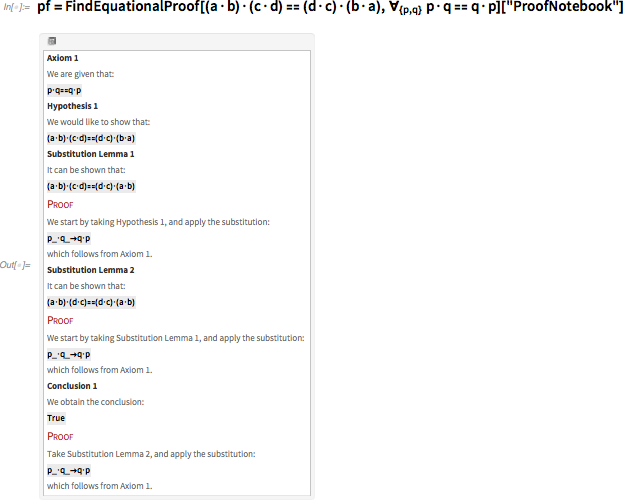✕ ```pf = FindEquationalProof[(a\[CenterDot]b)\[CenterDot](c\[CenterDot]d) \ == (d\[CenterDot]c)\[CenterDot](b\[CenterDot]a), \!\( \*SubscriptBox[\(\[ForAll]\), \({p, q}\)]\(p\[CenterDot]q == q\[CenterDot]p\)\)]["ProofNotebook"]```

Once one’s got a proof like this, it’s straightforward to just run through each of its steps, and check that they produce the result that’s claimed. But how does one find the proof? There are lots of different possible sequences of substitutions and transformations that one could do. So how does one find a sequence that successfully gets to the final result?

One might think: why not just try all possible sequences, and if there is any sequence that works, one will eventually find it? Well, the problem is that one quickly ends up with an astronomical number of possible sequences to check. And indeed the main art of automated theorem proving consists of finding ways to prune the number of sequences one has to check.

This quickly gets pretty technical, but the most important idea is easy to talk about if one knows basic algebra. Let’s say you’re trying to prove an algebraic result like: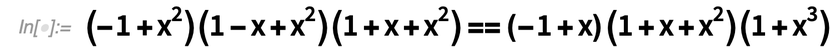✕ `(-1 + x^2) (1 - x + x^2) (1 + x + x^2) == (-1 + x) (1 + x + x^2) (1 + x^3)`

Well, there’s a guaranteed way to do this: just apply the rules of algebra to expand out each side—and immediately one can see they’re the same: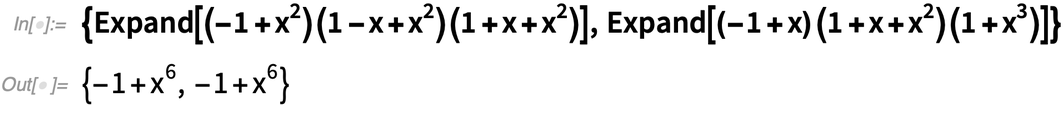✕ ```{Expand[(-1 + x^2) (1 - x + x^2) (1 + x + x^2)], Expand[(-1 + x) (1 + x + x^2) (1 + x^3)]}```

Why does this work? Well, it’s because there’s a way of taking algebraic expressions like this, and always systematically reducing them so that eventually they get to a standard form. OK, but so can one do the same thing for proofs with arbitrary axiom systems?

The answer is: not immediately. It works in algebra because algebra has a special property that guarantees one can always “make progress” in reducing expressions. But what was discovered independently several times in the 1970s (under names like the Knuth–Bendix and the Gröbner Basis algorithm) is that even if an axiom system doesn’t intrinsically have the appropriate property, one can potentially find “completions” of it that do.

And that’s what’s going on in typical proofs produced by FindEquationalProof (which is based on the Waldmeister (“master of trees”) system). There are so-called “critical pair lemmas” that don’t directly “make progress” themselves, but make it possible to set up paths that do. And the reason things get complicated is that even if the final expression one’s trying to get to is fairly short, one may have to go through all sorts of much longer intermediate expressions to get there. And so, for example, for the proof of the first Sheffer axiom above, here are the intermediate steps: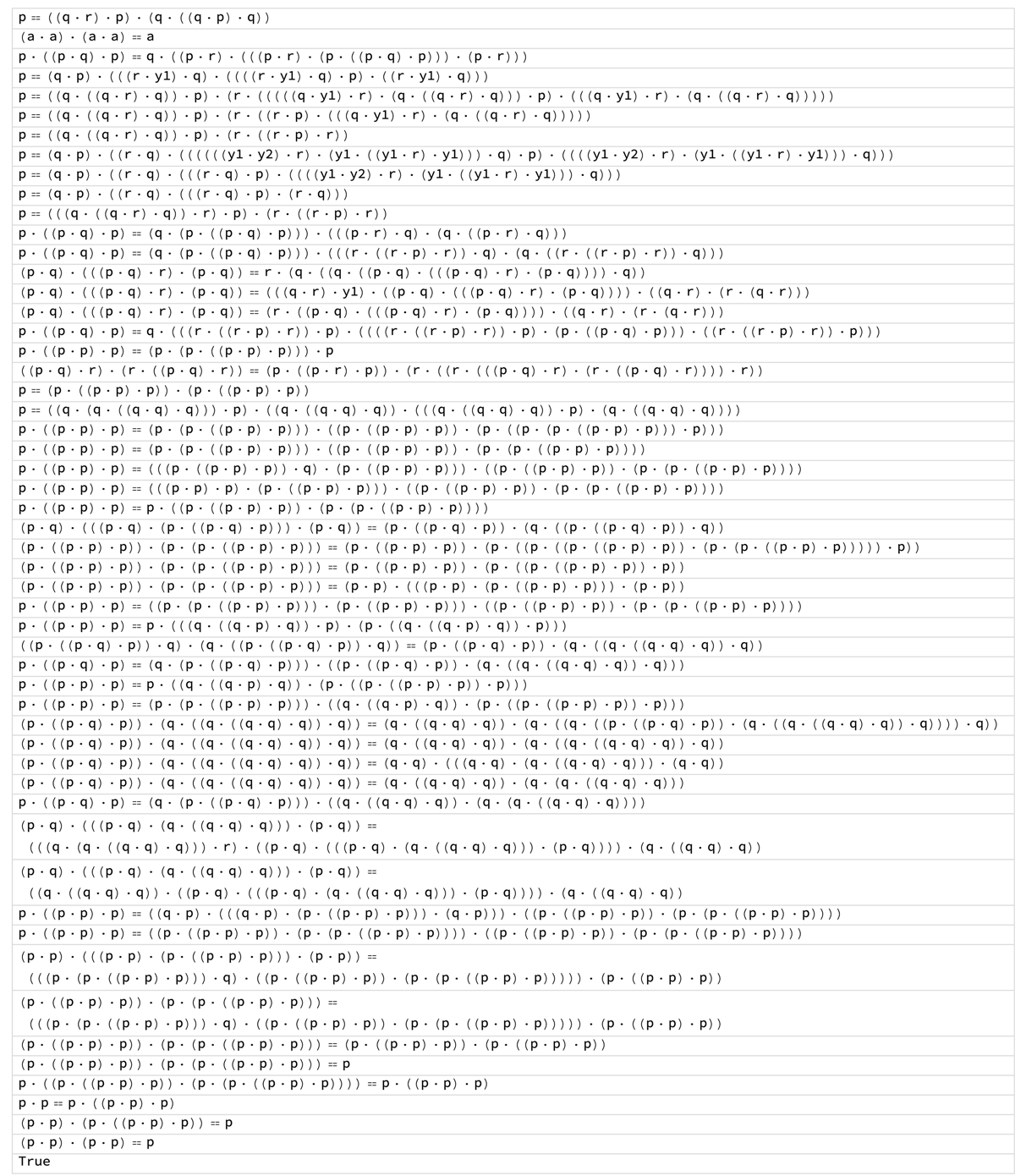In this case, the largest intermediate form is about 4 times the size of the original axiom. Here it is: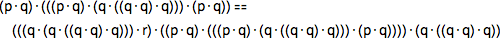✕ ```(p\[CenterDot]q)\[CenterDot](((p\[CenterDot]q)\[CenterDot](q\ \[CenterDot]((q\[CenterDot]q)\[CenterDot]q)))\[CenterDot](p\ \[CenterDot]q)) == (((q\[CenterDot](q\[CenterDot]((q\[CenterDot]q)\ \[CenterDot]q)))\[CenterDot]r)\[CenterDot]((p\[CenterDot]q)\ \[CenterDot](((p\[CenterDot]q)\[CenterDot](q\[CenterDot]((q\ \[CenterDot]q)\[CenterDot]q)))\[CenterDot](p\[CenterDot]q))))\ \[CenterDot](q\[CenterDot]((q\[CenterDot]q)\[CenterDot]q))```

One can represent expressions like this as a tree. Here’s this one, compared to the original axiom: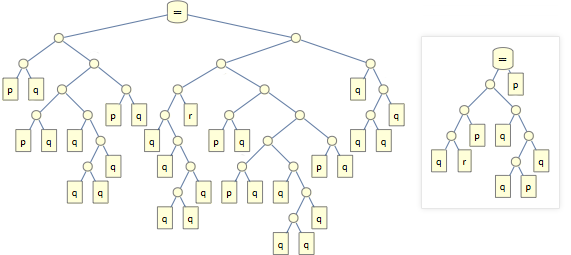And here’s how the sizes of intermediate steps evolve through the proofs found for each of the Sheffer axioms: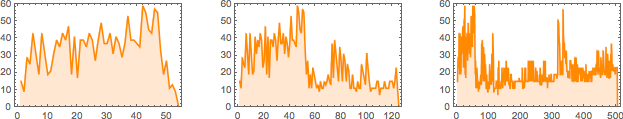## Why Is It So Hard?

Is it surprising that these proofs are so complicated? In some ways, not really. Because, after all, we know perfectly well that math can be hard. In principle it might have been that anything that’s true in math would be easy to prove. But one of the side effects of Gödel’s Theorem from 1931 was to establish that even things we can eventually prove can have proofs that are arbitrarily long.

And actually this is a symptom of the much more general phenomenon I call computational irreducibility. Consider a system governed, say, by the simple rule of a cellular automaton (and of course, every essay of mine must have a cellular automaton somewhere!). Now just run the system: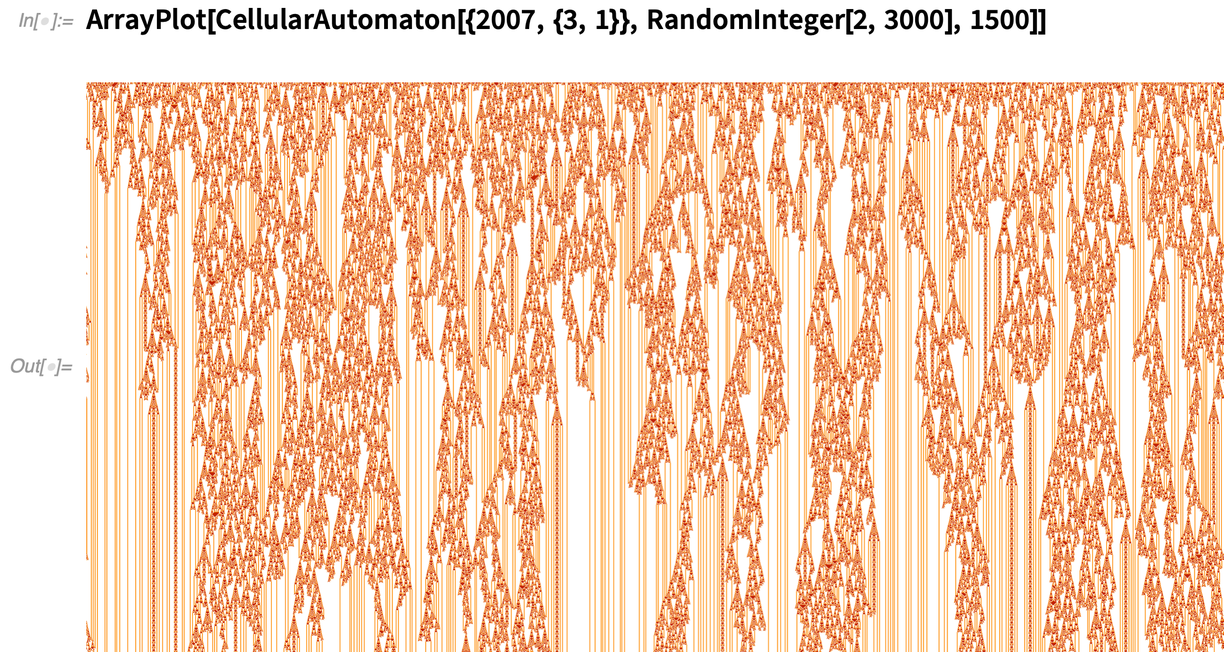✕ `ArrayPlot[CellularAutomaton[{2007,{3,1}},RandomInteger[2,3000],1500],Frame->False,ColorRules->{0->White,1->Hue[0.09, 1., 1.],2->Hue[0.03, 1., 0.76]}]`

One might have thought that given that there’s a simple rule that underlies the system, there’d always be a quick way to figure out what the system will do. But that’s not the case. Because according to my Principle of Computational Equivalence the operation of the system will often correspond to a computation that’s just as sophisticated as any computation that we could set up to figure out the behavior of the system. And this means that the actual behavior of the system in effect corresponds to an irreducible amount of computational work that we can’t in general shortcut in any way.

In the picture above, let’s say we want to know whether the pattern eventually dies out. Well, we could just keep running it, and if we’re lucky it’ll eventually resolve to something whose outcome is obvious. But in general there’s no upper bound to how far we’ll have to go to, in effect, prove what happens.

When we do things like the logic proofs above, it’s a slightly different setup. Instead of just running something according to definite rules, we’re asking whether there exists a way to get to a particular result by taking some series of steps that each follow a particular rule. And, yes, as a practical computational problem, this is immediately more difficult. But the core of the difficulty is still the same phenomenon of computational irreducibility—and that this phenomenon implies that there isn’t any general way to shortcut the process of working out what a system will do.

Needless to say, there are plenty of things in the world—especially in technology and scientific modeling, as well as in areas where there are various forms of regulation—that have traditionally been set up to implicitly avoid computational irreducibility, and to operate in ways whose outcome can readily be foreseen without an irreducible amount of computation.

But one of the implications of my Principle of Computational Equivalence is that this is a rather singular and contrived situation—because it says that computational irreducibility is in fact ubiquitous across systems in the computational universe.

OK, but what about mathematics? Maybe somehow the rules of mathematics are specially chosen to show computational reducibility. And there are indeed some cases where that’s true (and in some sense it even happens in logic). But for the most part it appears that the axiom systems of mathematics are not untypical of the space of all possible axiom systems—where computational irreducibility is inevitably rampant.

## What’s the Point of a Proof?

At some level, the point of a proof is to know that something is true. Of course, particularly in modern times, proof has very much taken a back seat to pure computation. Because in practice it’s much more common to want to generate things by explicit computation than it is to want to “go back” and construct a proof that something is true.

In pure mathematics, though, it’s fairly common to deal with things that at least nominally involve an infinite number of cases (“true for all primes”, etc.), for which at least direct computation can’t work. And when it comes to questions of verification (“can this program ever crash?” or “can this cryptocurrency ever get spent twice?”) it’s often more reasonable to attempt a proof than to do something like run all possible cases.

But in the actual practice of mathematics, there’s more to proof than just establishing if things are true. Back when Euclid first wrote his Elements, he just gave results, and proofs were “left to the reader”. But for better or worse, particularly over the past century, proof has become something that doesn’t just happen behind the scenes, but is instead actually the primary medium through which things are supposed to be communicated.

At some level I think it’s a quirk of history that proofs are typically today presented for humans to understand, while programs are usually just thought of as things for computers to run. Why has this happened? Well, at least in the past, proofs could really only be represented in essentially textual form—so if they were going to be used, it would have to be by humans. But programs have essentially always been written in some form of computer language. And for the longest time, that language tended to be set up to map fairly directly onto the low-level operations of the computer—which meant that it was readily “understandable” by the computer, but not necessarily by humans.

But as it happens, one of the main goals of my own efforts over the past several decades has been to change this—and to develop in the Wolfram Language a true “computational communication language” in which computational ideas can be communicated in a way that is readily understandable to both computers and humans.

There are many consequences of having such a language. But one of them is that it changes the role of proof. Let’s say one’s looking at some mathematical result. Well, in the past the only plausible way to communicate how one should understand it was to give a proof that people could read. But now something different is possible: one can give a Wolfram Language program that computes the result. And in many ways this is a much more powerful way to communicate why the result is true. Because every piece of the program is something precise and unambiguous—that if one wants to, one can actually run. There’s no issue of trying to divine what some piece of text means, perhaps filling in some implicit assumptions. Instead, everything is right there, in absolutely explicit form.

OK, so what about proof? Are there in fact unambiguous and precise ways to write proofs? Well, potentially yes, though it’s not particularly easy. And even though the main Wolfram Language has now existed for 30 years, it’s taken until pretty much now to figure out a reasonable way to represent in it even such structurally comparatively straightforward proofs as the one for my axiom system above.

One can imagine authoring proofs in the Wolfram Language much like one authors programs—and indeed we’re working on seeing how to provide high-level versions of this kind of “proof assistant” functionality. But the proof of my axiom system that I showed above is not something anyone authored; it’s something that was found by the computer. And as such, it’s more like the output of running a program than like a program itself. (Like a program, though, the proof can in some sense be “run” to verify the result.)

## Generating Understandability

Most of the time when people use the Wolfram Language—or Wolfram|Alpha—they just want to compute things. They’re interested in getting results, not in understanding why they get the results they do. But in Wolfram|Alpha, particularly in areas like math and chemistry, a popular feature for students is “step-by-step solutions”: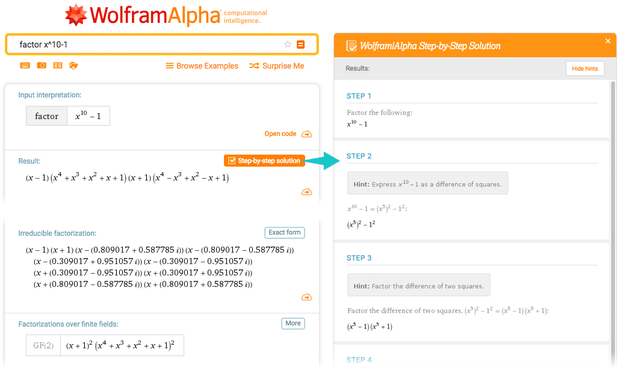When Wolfram|Alpha does something like computing an integral, it’s using all sorts of powerful systematic algorithmic techniques optimized for getting answers. But when it’s asked to show steps it needs to do something different: it needs instead to explain step by step why it gets the result it does.

It wouldn’t be useful for it to explain how it actually got the result; it’s a very non-human process. Instead, it basically has to figure out how the kinds of operations humans learn can be used to get the result. Often it’ll figure out some trick that can be used. Yes, there’ll be a systematic way to do it that’ll always work. But it involves too many “mechanical” steps. The “trick” (“trig substitution”, “integration by parts”, whatever) won’t work in general, but in this particular case it’ll provide a faster way to get to the answer.

OK, but what about getting understandable versions of other things? Like the operation of programs in general. Or like the proof of my axiom system.

Let’s start by talking about programs. Let’s say one’s written a program, and one wants to explain how it works. One traditional approach is just to “include comments” in the code. Well, if one’s writing in a traditional low-level language, that may be the best one can do. But the whole point of the Wolfram Language being a computational communication language is that the language itself is supposed to allow you to communicate ideas, without needing extra pieces of English text.

It takes effort to make a Wolfram Language program be a good piece of exposition, just like it takes effort to make English text a good piece of exposition. But one can end up with a piece of Wolfram Language code that really explains very clearly how it works just through the code itself.

Of course, it’s very common for the actual execution of the code to do things that can’t readily be foreseen just from the program. I’ll talk about extreme cases like cellular automata soon. But for now let’s imagine that one’s constructed a program where there’s some ability to foresee the broad outlines of what it does.

And in such a case, I’ve found that computational essays (presented as Wolfram Notebooks) are a great tool in explaining what’s going on. It’s crucial that the Wolfram Language is symbolic, so it’s possible to run even the tiniest fragments of any program on their own (with appropriate symbolic expressions as input or output). And when one does this, one can present a succession of steps in the program as a succession of elements in the dialog that forms the core of a computational notebook.

In practice, it’s often critical to create visualizations of inputs or outputs. Yes, everything can be represented as an explicit symbolic expression. But we humans often have a much easier time understanding things when they’re presented visually, rather than as some kind of one-dimensional language-like string.

Of course, there’s something of an art to creating good visualizations. But in the Wolfram Language we’ve managed to go a long way towards automating this art—often using pretty sophisticated machine learning and other algorithms to do things like lay out networks or graphics elements.

What about just starting from the raw execution trace for a program? Well, it’s hard. I’ve done experiments on this for decades, and never been very satisfied with the results. Yes, you can zoom in to see lots of details of what’s going on. But when it comes to knowing the “big picture” I’ve never found any particularly good techniques for automatically producing things that are terribly useful.

At some level it’s similar to the general problem of reverse engineering. You are shown some final machine code, chip design, or whatever. But now you want to go backwards to reconstruct the higher-level description that some human started from, that was somehow “compiled” to what you see.

In the traditional approach to engineering, where one builds things up incrementally, always somehow being able to foresee the consequences of what one’s building, this approach can in principle work. But if one does engineering by just searching the computational universe to find an optimal program (much like I searched possible axiom systems to find one for logic), then there’s no guarantee that there’s any “human story” or explanation behind this program.

It’s a similar problem in natural science. You see some elaborate set of things happening in some biological system. Can one “reverse engineer” these to find an “explanation” for them? Sometimes one might be able to say, for example, that evolution by natural selection would be likely to lead to something. Or that it’s just common in the computational universe and so is likely to occur. But there’s no guarantee that the natural world is set up in any way that necessarily allows human explanation.

Needless to say, when one makes models for things, one inevitably considers only the particular aspects that one’s interested in, and idealizes everything else away. And particularly in areas like medicine, it’s not uncommon to end up with some approximate model that’s a fairly shallow decision tree that’s easy to explain, at least as far as it goes.

## The Nature of Explainability

What does it mean to say that something is explainable? Basically it’s that humans can understand it.

So what does it take for humans to understand something? Well, somehow we have to be able to “wrap our brains around it”. Let’s take a typical cellular automaton with complex behavior. A computer has no problem following each step in the evolution. And with immense effort a human could laboriously reproduce what a computer does.

But one wouldn’t say that means the human “understands” what the cellular automaton is doing. To get to that point, the human would have to be readily able to reason about how the cellular automaton behaves, at some high level. Or put another way, the human would have to be able to “tell a story” that other humans could readily understand, about how the cellular automaton behaves.

Is there a general way to do this? Well, no, because of computational irreducibility. But it can still be the case that certain features that humans choose to care about can be explained in some reduced, higher-level way.

How does this work? Well, in a sense it requires that some higher-level language be constructed that can describe the features one’s interested in. Looking at a typical cellular automaton pattern, one might try to talk not in terms of colors of huge numbers of individual cells, but instead in terms of the higher-level structures one can pick out. And the key point is that it’s possible to make at least a partial catalog of these structures: even though there are lots of details that don’t quite fit, there are still particular structures that occur often.

And if we were going to start “explaining” the behavior of the cellular automaton, we’d typically begin by giving the structures names, and then we’d start talking about what’s going on in terms of these named things.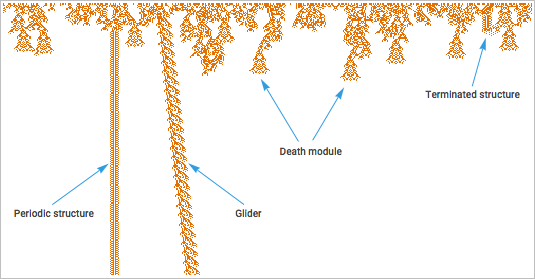The case of a cellular automaton has an interesting simplifying feature: because it operates according to simple deterministic rules, there are structures that just repeat identically. If we’re dealing with things in the natural world, for example, we typically won’t see this kind of identical repetition. Instead, it’ll just be that this tiger, say, is extremely similar to this other one, so we can call them both “tigers”, even though their atoms are not identical in their arrangement.

What’s the bigger picture of what’s going on? Well, it’s basically that we’re using the idea of symbolic representation. We’re saying that we can assign something—often a word—that we can use to symbolically describe a whole class of things, without always having to talk about all the detailed parts of each thing.

In effect it’s a kind of information compression: we’re using symbolic constructs to find a shorter way to describe what we’re interested in.

Let’s imagine we’ve generated a giant structure, say a mathematical one: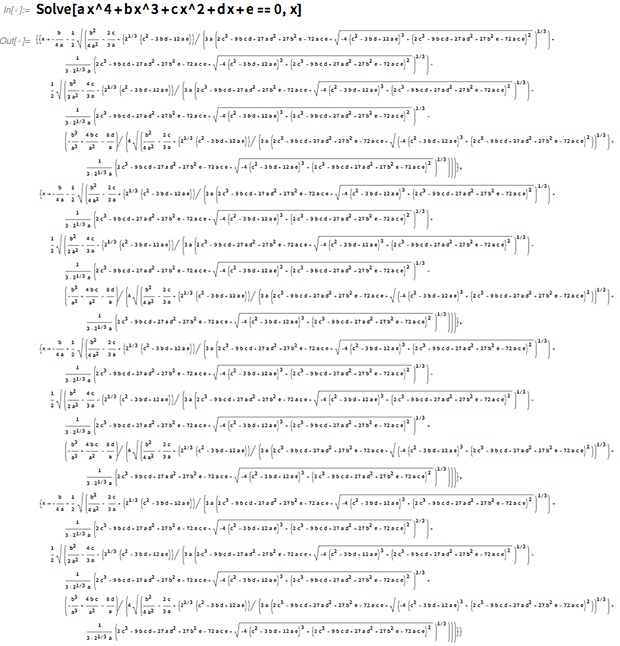✕ `Solve[a x^4 + b x^3 + c x^2 + d x + e == 0, x]`

Well, a first step is to generate a kind of internal higher-level representation. For example, we might find substructures that appear repeatedly. And we might then assign names to them. And then display a “skeleton” of the whole structure in terms of these names: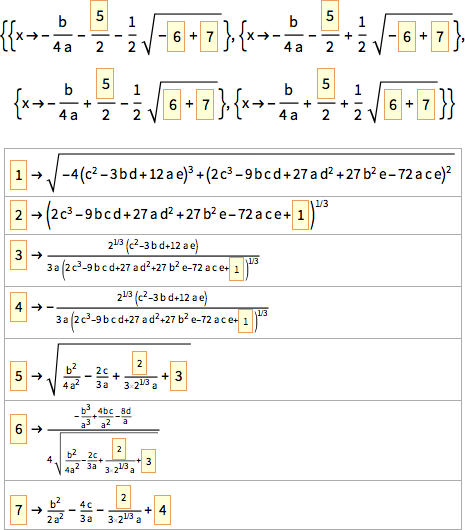And, yes, this kind of “dictionary compression”–like scheme is useful in bringing a first level of explainability.

But let’s go back to the proof of my axiom system. The lemmas that were generated in this proof are precisely set up to be elements that are used repeatedly (a bit like shared common subexpressions). But even having in effect factored them out, we’re still left with a proof that is not something that we humans can readily understand.

So how can we go further? Well, basically we have to come up with some yet-higher-level description. But what might this be?

## The Concept of Concepts

If you’re trying to explain something to somebody, it’s a lot easier when there’s something similar that they’ve already understood. Imagine trying to explain a modern drone to someone from the Stone Age. It’d probably be pretty difficult. But explaining it to someone from 50 years ago, who’d already seen helicopters and model airplanes etc., would be a lot easier.

And ultimately the point is that when we explain something, we do it in some language that both we and whoever we’re explaining it to know. And the richer this language is, the fewer new elements we have to introduce in order to communicate whatever it is that we’re trying to explain.

There’s a pattern that’s been repeated throughout intellectual history. Some particular collection of things gets seen a bunch of times. And gradually it’s understood that these things are all somehow abstractly similar. And they can all be described in terms of some particular new concept, often referred to by some new word or phrase.

Let’s say one had seen things like water and blood and oil. Well, at some point one realizes that there’s a general concept of “liquid”, and all of these can be described as liquids. And once one has this concept, one can start reasoning in terms of it, and identifying more concepts—like, say, viscosity—that build on it.

When does it makes sense to group things into a concept? Well, that’s a difficult question, which can’t ultimately be answered without foreseeing everything that might be done with that concept. And in practice, in the evolution of human language and human ideas there’s some kind of process of progressive approximation that goes on.

There’s a much more rapid recapitulation that happens in a modern machine learning system. Imagine taking all sorts of objects that one’s seen in the world, and just feeding them to FeatureSpacePlot and seeing what comes out. Well, if one gets definite clusters in feature space, then one might reasonably think that each of these clusters should be identified as corresponding to a “concept”, that we could for example label with a word.

Now, to be fair, what’s happening with FeatureSpacePlot—like in human intellectual development—is in some ways incremental. Because to lay the objects out in feature space, FeatureSpacePlot is using features that it’s learned how to extract from previous categorizations it knows about.

But, OK, given the world as it is, what are the best categories—or best concepts—one can use to describe things? Well, it’s an evolving story. And in fact breakthroughs—whether in science, technology or elsewhere—are very often precisely associated with the realization that some new category or concept can usefully be identified.

But in the actual evolution of our civilization, there’s a kind of spiral at work. First some particular concept is identified—say the idea of a program. And once some concept has been identified, people start using it, and thinking in terms of it. And pretty soon all sorts of new things have been constructed on the basis of that concept. But then another level of abstraction is identified, and new concepts get constructed, building on top of the previous one.

It’s pretty much the same story for the technology stack of modern civilization, and its “intellectual stack”. Both involve towers of concepts, and successive levels of abstraction.

## The Problem of Education

In order for people to be able to communicate using some concept, they have to have learned about it. And, yes, there are some concepts (like object permanence) that humans automatically learn by themselves just by observing the natural world. But looking for example at a list of common words in modern English, it’s pretty clear that most of the concepts that we now use in modern civilization aren’t ones that people can just learn for themselves from the natural world.

Instead—much like a modern machine learning system—at the very least they need some “specially curated” experience of the world, organized to highlight particular concepts. And for more abstract areas (like mathematics) they probably need explicit exposure to the concepts themselves in their raw abstract forms.

But, OK, as the “intellectual stack” of civilization advances, will we always have to learn progressively more? We might worry that at some point our brains just won’t be able to keep up, and we’d have to add some kind of augmentation. But perhaps fortunately, I think it’s one of those cases where the problem can instead most likely be “solved in software”.

The issue is this: At any given point in history, there’s a certain set of concepts that are important in being able to operate in the world as it is at that time. And, yes, as civilization progresses new things are discovered, and new concepts are introduced. But there’s another process at work as well: new concepts bring new levels of abstraction, which typically subsume large numbers of earlier concepts.

We often see this in technology. There was a time when to operate a computer you needed to know all sorts of low-level details. But over time those got abstracted away, so all you need to know is some general concept. You click an icon and things start to happen—and you don’t have to understand operating systems, or interrupt handlers or schedulers, or any of those details.

Needless to say, the Wolfram Language provides a great example of all this. Because it goes to tremendous trouble to “automate out” lots of low-level details (for example about what specific algorithm to use) and let human users just think about things in terms of higher-level concepts.

Yes, there still need to be some people who understand the details “underneath” the abstraction (though I’m not sure how many flint knappers modern society needs). But mostly education can concentrate on teaching at a higher level.

There’s often an implicit assumption in education that to reach higher-level concepts one has to somehow recapitulate the history of how those concepts were historically arrived at. But usually—and perhaps always—this doesn’t seem to be true. In an extreme case, one might imagine that to teach about computers, one would have to recapitulate the history of mathematical logic. But actually we know that people can go straight to modern concepts of computing, without recapitulating any of the history.

But what is ultimately the understandability network of concepts? Are there concepts that can only be understood if one already understands other concepts? Given a particular ambient experience for a human (or particular background training for a neural network) there is presumably some ordering.

But I suspect that something analogous to computational universality probably implies that if one’s just dealing with a “raw brain” then one could start anywhere. So if some alien were exposed to category theory and little else from the very beginning, they’d no doubt build a network of concepts where this is at the root, and maybe what for us is basic arithmetic would be something only reached in their analog of math graduate school.

Of course, such an alien might form their technology stack and their built environment in a quite different way from us—much as the recent history of our own civilization might have been very different if computers had successfully been developed in the 1800s rather than in the mid-1900s.

## The Progress of Mathematics

I’ve often wondered to what extent the historical trajectory of human mathematics is an “accident”, and to what extent it’s somehow inexorable. As I mentioned earlier, at the level of formal systems there are many possible axiom systems from which one could construct something that is formally like mathematics.

But the actual history of mathematics did not start with arbitrary axiom systems. It started—in Babylonian times—with efforts to use arithmetic for commerce and geometry for land surveying. And from these very practical origins, successive layers of abstraction have been added that have led eventually to modern mathematics—with for example numbers being generalized from positive integers, to rationals, to roots, to all integers, to decimals, to complex numbers, to algebraic numbers, to quaternions and so on.

Is there an inexorability to this progression of abstraction? I suspect to some extent there is. And probably it’s a similar story as with other kinds of concept formation. Given some stage that’s been reached, there are various things that can readily get studied, and after a while groups of them are seen to be examples of more general and abstract constructs—which then in turn define another stage from which new things can be studied.

Are there ways to break out of this cycle? One possibility would be through doing experiments in mathematics. Yes, one can systematically prove things about particular mathematical systems. But one can also just empirically notice mathematical facts—like Ramanujan’s observation that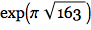is numerically close to an integer. And the question is: are things like this just “random facts of mathematics” or do they somehow fit into the whole “fabric of mathematics”?

One can ask the same kind of thing about questions in mathematics. Is the question of whether odd perfect numbers exist (which has been unanswered since Pythagoras) a core question in mathematics, or is it, in a sense, a random question that doesn’t connect into the fabric of mathematics?

Just like one can enumerate things like axiom systems, so also one can imagine enumerating possible questions in mathematics. But if one does this, I suspect there’s immediately an issue. Gödel’s Theorem establishes that in axiom systems like the one for arithmetic there are “formally undecidable” propositions, that can’t be proved or disproved from within the axiom system.

But the particular examples that Gödel constructed seemed far from anything that would arise naturally in doing mathematics. And for a long time it was assumed that somehow the phenomenon of undecidability was something that, while in principle present, wasn’t going to be relevant in “real mathematics”.

However, with my Principle of Computational Equivalence and my experience in the computational universe, I’ve come to the strong conclusion that this isn’t correct—and that instead undecidability is actually close at hand even in typical mathematics as it’s been practiced. Indeed, I won’t be surprised if a fair fraction of the current famous unsolved problems of mathematics (Riemann Hypothesis, P=NP, etc.) actually turn out to be in effect undecidable.

But if there’s undecidability all around, how come there’s so much mathematics that’s successfully been done? Well, I think it’s because the things that have been done have implicitly been chosen to avoid undecidability, basically just by virtue of the way mathematics has been built up. Because if what one’s doing is basically to form progressive levels of abstraction based on things one has shown are true, one’s basically setting up a path that’s going to be able to move forward without being forced into undecidability.

Of course, doing experimental mathematics or asking “random questions” may immediately land one in some area that’s full of undecidability. But at least so far in its history, this hasn’t been the way the mainstream discipline of mathematics has evolved.

So what about those “random facts of mathematics”? Well, it’s pretty much like in other areas of intellectual endeavor. “Random facts” don’t really get integrated into a line of intellectual development until some structure—and typically some abstract concepts—are built around them.

My own favorite discoveries about the origins of complexity in systems like the rule 30 cellular automaton are a good example. Yes, similar phenomena had been seen—even millennia earlier (think: randomness in the sequence of primes). But without a broader conceptual framework nobody really paid attention to them.

Nested patterns are another example. There are isolated examples of these in mosaics from the 1200s, but nobody really paid attention to them until the whole framework around nesting and fractals emerged in the 1980s.

It’s the same story over and over again: until abstract concepts around them have been identified, it’s hard to really think about new things, even when one encounters phenomena that exhibit them.

And so, I suspect, it is with mathematics: there’s a certain inevitable layering of abstract concept on top of abstract concept that defines the trajectory of mathematics. Is it a unique path? Undoubtedly not. In the vast space of possible mathematical facts, there are particular directions that get picked, and built along. But others could have been picked instead.

So does this mean that the subject matter of mathematics is inevitably dominated by historical accidents? Not as much as one might think. Because—as mathematics has discovered over and over again, starting with things like algebra and geometry—there’s a remarkable tendency for different directions and different approaches to wind up having equivalences or correspondences in the end.

And probably at some level this is a consequence of the Principle of Computational Equivalence, and the phenomenon of computational universality: even though the underlying rules (or underlying “language”) used in different areas of mathematics are different, there ends up being some way to translate between them—so that at the next level of abstraction the path that was taken no longer critically matters.

## The Logic Proof and the Automation of Abstraction

OK, so let’s go back to the logic proof. How does it connect to typical mathematics? Well, right now, it basically doesn’t. Yes, the proof has the same nominal form as a standard mathematical proof. But it isn’t “human-mathematician friendly”. It’s all just mechanical details. It doesn’t connect to higher-level abstract concepts that a human mathematician can readily understand.

It would help a lot if we discovered that nontrivial lemmas in the proof already appeared in the mathematics literature. (I don’t think any of them do, but our theorem-searching capabilities haven’t gotten to the point where one can be sure.) But if they did appear, then this would likely give us a way to connect these lemmas to other things in mathematics, and in effect to identify a circle of abstract concepts around them.

But without that, how can the proof become explainable?

Well, maybe there’s just a different way to do the proof that’s fundamentally more connected to existing mathematics. But even given the proof as we have it now, one could imagine “building out” new concepts that would define a higher level of abstraction and put the proof in a more general context.

I’m not sure how to do either of these things. I’ve considered sponsoring a prize (analogous to my 2007 Turing machine prize) for “making the proof explainable”. But it’s not at all clear how one could objectively judge “explainability”. (Maybe one could ask for a 1-hour video that would successfully explain the proof to a typical mathematician—but this is definitely rather subjective.)

But just like we can automate things like finding aesthetic layouts for networks, perhaps we can automate the process of making a proof explainable. The proof as it is right now basically just says (without explanation), “Consider these few hundred lemmas”. But let’s say we could identify a modest number of “interesting” lemmas. Maybe we could somehow add these to our canon of known mathematics and then be able to use them to understand the proof.

There’s an analogy here with language design. In building up the Wolfram Language what I’ve basically done is to try to identify “lumps of computational work” that people will often want. Then we make these into built-in functions in the language, with particular names that people can use to refer to them.

A similar process goes on—though in a much less organized way—in the evolution of human natural languages. “Lumps of meaning” that turn out to be useful eventually get represented by words in the language. Sometimes they start as phrases constructed out of a few existing words. But the most impactful ones are typically sufficiently far away from anything that has come before that they just arrive as new words with potentially quite-hard-to-give definitions.

In the design of the Wolfram Language—with functions named with English words—I leverage the “ambient understanding” that comes from the English words (and sometimes from their meanings in common applications of computation).

One would want to do something similar in identifying lemmas to add to our canon of mathematics. Not only would one want to make sure that each lemma was somehow “intrinsically interesting”, but one would also want when possible to select lemmas that are “easy to reach” from existing known mathematical results and concepts.

But what does it mean for a lemma to be “intrinsically interesting”? I have to say that before I worked on A New Kind of Science, I assumed that there was great arbitrariness and historical accident in the choice of lemmas (or theorems) in any particular areas of mathematics that get called out and given names in typical textbooks.

But when I looked in detail at theorems in basic logic, I was surprised to find something different. Let’s say one arranges all the true theorems of basic logic in order of their sizes (e.g. p = p might come first; p AND p = p a bit later, and so on). When one goes through this list there’s lots of redundancy. Indeed, most of the theorems end up being trivial extensions of theorems that have already appeared in the list.

But just sometimes one gets to a theorem that essentially gives new information—and that can’t be proved from the theorems that have already appeared in the list. And here’s the remarkable fact: there are 14 such theorems, and they essentially correspond exactly with the theorems that are typically given names in textbooks of logic. (Here AND is ∧, OR is ∨, and NOT is ¬.)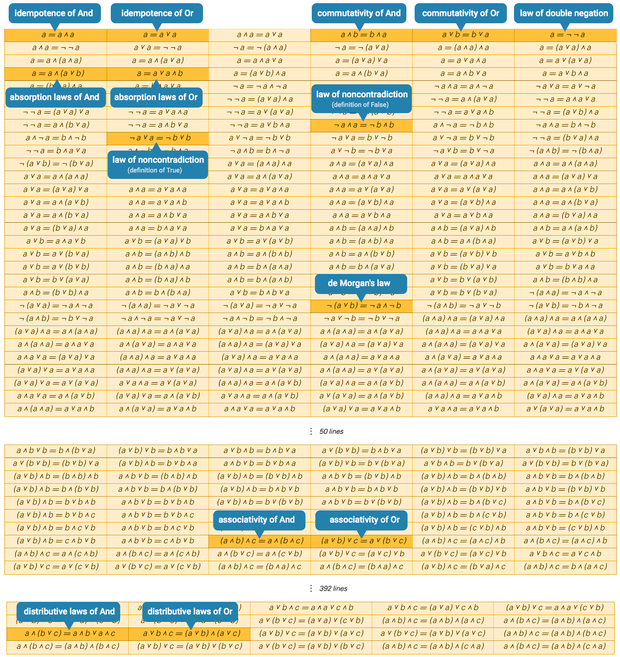In other words, at least in this case, the named or “interesting” theorems are the ones that give minimal statements of new information. (Yes, after a while, by this definition there will be no new information, because one will have encountered all the axioms needed to prove anything that can be proved—though one can go a bit further with this approach by starting to discuss limiting the complexity of proofs that are allowed.)

What about with NAND theorems, like the ones in the proof? Once again, one can arrange all true NAND theorems in order—and then find which of them can’t be proved from any earlier in the list: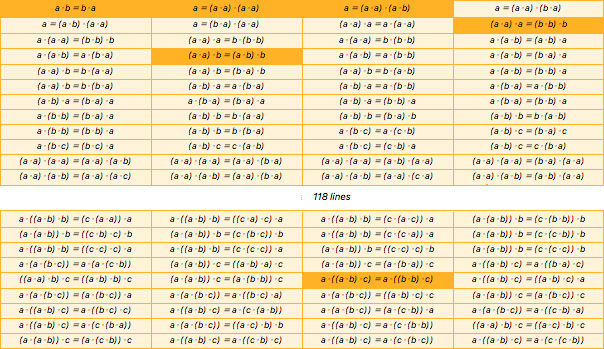✕ `{{a\[CenterDot]b==b\[CenterDot]a,a==(a\[CenterDot]a)\[CenterDot](a\[CenterDot]a),a==(a\[CenterDot]a)\[CenterDot](a\[CenterDot]b),(a\[CenterDot]a)\[CenterDot]a==(b\[CenterDot]b)\[CenterDot]b,(a\[CenterDot]a)\[CenterDot]b==(a\[CenterDot]b)\[CenterDot]b,a\[CenterDot]((a\[CenterDot]b)\[CenterDot]c)==a\[CenterDot]((b\[CenterDot]b)\[CenterDot]c)},{a\[CenterDot]b==b\[CenterDot]a,a==(a\[CenterDot]a)\[CenterDot](a\[CenterDot]a),a==(a\[CenterDot]a)\[CenterDot](a\[CenterDot]b),a==(a\[CenterDot]a)\[CenterDot](b\[CenterDot]a),a==(a\[CenterDot]b)\[CenterDot](a\[CenterDot]a),a==(b\[CenterDot]a)\[CenterDot](a\[CenterDot]a),(a\[CenterDot]a)\[CenterDot]a==a\[CenterDot](a\[CenterDot]a),(a\[CenterDot]a)\[CenterDot]a==(b\[CenterDot]b)\[CenterDot]b,a\[CenterDot](a\[CenterDot]a)==(b\[CenterDot]b)\[CenterDot]b,(a\[CenterDot]a)\[CenterDot]a==b\[CenterDot](b\[CenterDot]b),a\[CenterDot](a\[CenterDot]a)==b\[CenterDot](b\[CenterDot]b),a\[CenterDot](a\[CenterDot]b)==(a\[CenterDot]b)\[CenterDot]a,a\[CenterDot](a\[CenterDot]b)==a\[CenterDot](b\[CenterDot]a),(a\[CenterDot]a)\[CenterDot]b==(a\[CenterDot]b)\[CenterDot]b,a\[CenterDot](a\[CenterDot]b)==a\[CenterDot](b\[CenterDot]b),a\[CenterDot](a\[CenterDot]b)==(b\[CenterDot]a)\[CenterDot]a,(a\[CenterDot]a)\[CenterDot]b==b\[CenterDot](a\[CenterDot]a),(a\[CenterDot]a)\[CenterDot]b==(b\[CenterDot]a)\[CenterDot]b,(a\[CenterDot]a)\[CenterDot]b==b\[CenterDot](a\[CenterDot]b),a\[CenterDot](a\[CenterDot]b)==(b\[CenterDot]b)\[CenterDot]a,(a\[CenterDot]a)\[CenterDot]b==b\[CenterDot](b\[CenterDot]a),(a\[CenterDot]b)\[CenterDot]a==a\[CenterDot](b\[CenterDot]a),(a\[CenterDot]b)\[CenterDot]a==a\[CenterDot](b\[CenterDot]b),a\[CenterDot](b\[CenterDot]a)==a\[CenterDot](b\[CenterDot]b),(a\[CenterDot]b)\[CenterDot]a==(b\[CenterDot]a)\[CenterDot]a,a\[CenterDot](b\[CenterDot]a)==(b\[CenterDot]a)\[CenterDot]a,(a\[CenterDot]b)\[CenterDot]a==(b\[CenterDot]b)\[CenterDot]a,a\[CenterDot](b\[CenterDot]a)==(b\[CenterDot]b)\[CenterDot]a,a\[CenterDot](b\[CenterDot]b)==(b\[CenterDot]a)\[CenterDot]a,(a\[CenterDot]b)\[CenterDot]b==b\[CenterDot](a\[CenterDot]a),(a\[CenterDot]b)\[CenterDot]b==(b\[CenterDot]a)\[CenterDot]b,(a\[CenterDot]b)\[CenterDot]b==b\[CenterDot](a\[CenterDot]b),a\[CenterDot](b\[CenterDot]b)==(b\[CenterDot]b)\[CenterDot]a,(a\[CenterDot]b)\[CenterDot]b==b\[CenterDot](b\[CenterDot]a),a\[CenterDot](b\[CenterDot]c)==a\[CenterDot](c\[CenterDot]b),(a\[CenterDot]b)\[CenterDot]c==(b\[CenterDot]a)\[CenterDot]c,a\[CenterDot](b\[CenterDot]c)==(b\[CenterDot]c)\[CenterDot]a,(a\[CenterDot]b)\[CenterDot]c==c\[CenterDot](a\[CenterDot]b),a\[CenterDot](b\[CenterDot]c)==(c\[CenterDot]b)\[CenterDot]a,(a\[CenterDot]b)\[CenterDot]c==c\[CenterDot](b\[CenterDot]a),(a\[CenterDot]a)\[CenterDot](a\[CenterDot]a)==(a\[CenterDot]a)\[CenterDot](a\[CenterDot]b),(a\[CenterDot]a)\[CenterDot](a\[CenterDot]a)==(a\[CenterDot]a)\[CenterDot](b\[CenterDot]a),(a\[CenterDot]a)\[CenterDot](a\[CenterDot]a)==(a\[CenterDot]b)\[CenterDot](a\[CenterDot]a),(a\[CenterDot]a)\[CenterDot](a\[CenterDot]a)==(b\[CenterDot]a)\[CenterDot](a\[CenterDot]a),(a\[CenterDot]a)\[CenterDot](a\[CenterDot]b)==(a\[CenterDot]a)\[CenterDot](a\[CenterDot]c),(a\[CenterDot]a)\[CenterDot](a\[CenterDot]b)==(a\[CenterDot]a)\[CenterDot](b\[CenterDot]a),(a\[CenterDot]a)\[CenterDot](a\[CenterDot]b)==(a\[CenterDot]a)\[CenterDot](c\[CenterDot]a),(a\[CenterDot]a)\[CenterDot](a\[CenterDot]b)==(a\[CenterDot]b)\[CenterDot](a\[CenterDot]a)},{a\[CenterDot]((a\[CenterDot]b)\[CenterDot]b)==(c\[CenterDot](a\[CenterDot]a))\[CenterDot]a,a\[CenterDot]((a\[CenterDot]b)\[CenterDot]b)==((c\[CenterDot]a)\[CenterDot]c)\[CenterDot]a,a\[CenterDot]((a\[CenterDot]b)\[CenterDot]b)==(c\[CenterDot](a\[CenterDot]c))\[CenterDot]a,(a\[CenterDot](a\[CenterDot]b))\[CenterDot]b==(c\[CenterDot](b\[CenterDot]b))\[CenterDot]b,(a\[CenterDot](a\[CenterDot]b))\[CenterDot]b==((c\[CenterDot]b)\[CenterDot]c)\[CenterDot]b,(a\[CenterDot](a\[CenterDot]b))\[CenterDot]b==(c\[CenterDot](b\[CenterDot]c))\[CenterDot]b,a\[CenterDot]((a\[CenterDot]b)\[CenterDot]b)==(c\[CenterDot](c\[CenterDot]a))\[CenterDot]a,(a\[CenterDot](a\[CenterDot]b))\[CenterDot]b==(c\[CenterDot](c\[CenterDot]b))\[CenterDot]b,a\[CenterDot]((a\[CenterDot]b)\[CenterDot]b)==((c\[CenterDot]c)\[CenterDot]c)\[CenterDot]a,a\[CenterDot]((a\[CenterDot]b)\[CenterDot]b)==(c\[CenterDot](c\[CenterDot]c))\[CenterDot]a,(a\[CenterDot](a\[CenterDot]b))\[CenterDot]b==((c\[CenterDot]c)\[CenterDot]c)\[CenterDot]b,(a\[CenterDot](a\[CenterDot]b))\[CenterDot]b==(c\[CenterDot](c\[CenterDot]c))\[CenterDot]b,a\[CenterDot](a\[CenterDot](b\[CenterDot]c))==a\[CenterDot](a\[CenterDot](c\[CenterDot]b)),(a\[CenterDot](a\[CenterDot]b))\[CenterDot]c==((a\[CenterDot]b)\[CenterDot]a)\[CenterDot]c,(a\[CenterDot](a\[CenterDot]b))\[CenterDot]c==(a\[CenterDot](b\[CenterDot]a))\[CenterDot]c,a\[CenterDot]((a\[CenterDot]b)\[CenterDot]c)==a\[CenterDot]((b\[CenterDot]a)\[CenterDot]c),((a\[CenterDot]a)\[CenterDot]b)\[CenterDot]c==((a\[CenterDot]b)\[CenterDot]b)\[CenterDot]c,(a\[CenterDot](a\[CenterDot]b))\[CenterDot]c==(a\[CenterDot](b\[CenterDot]b))\[CenterDot]c,a\[CenterDot]((a\[CenterDot]b)\[CenterDot]c)==a\[CenterDot]((b\[CenterDot]b)\[CenterDot]c),a\[CenterDot]((a\[CenterDot]b)\[CenterDot]c)==((a\[CenterDot]b)\[CenterDot]c)\[CenterDot]a,a\[CenterDot](a\[CenterDot](b\[CenterDot]c))==(a\[CenterDot](b\[CenterDot]c))\[CenterDot]a,a\[CenterDot](a\[CenterDot](b\[CenterDot]c))==a\[CenterDot]((b\[CenterDot]c)\[CenterDot]a),a\[CenterDot](a\[CenterDot](b\[CenterDot]c))==((a\[CenterDot]b)\[CenterDot]c)\[CenterDot]c,(a\[CenterDot](a\[CenterDot]b))\[CenterDot]c==(a\[CenterDot](b\[CenterDot]c))\[CenterDot]c,a\[CenterDot]((a\[CenterDot]b)\[CenterDot]c)==a\[CenterDot]((b\[CenterDot]c)\[CenterDot]c),a\[CenterDot]((a\[CenterDot]b)\[CenterDot]c)==a\[CenterDot](c\[CenterDot](a\[CenterDot]b)),a\[CenterDot](a\[CenterDot](b\[CenterDot]c))==(a\[CenterDot](c\[CenterDot]b))\[CenterDot]a,a\[CenterDot](a\[CenterDot](b\[CenterDot]c))==a\[CenterDot]((c\[CenterDot]b)\[CenterDot]a),a\[CenterDot]((a\[CenterDot]b)\[CenterDot]c)==a\[CenterDot](c\[CenterDot](b\[CenterDot]a)),a\[CenterDot](a\[CenterDot](b\[CenterDot]c))==((a\[CenterDot]c)\[CenterDot]b)\[CenterDot]b,a\[CenterDot]((a\[CenterDot]b)\[CenterDot]c)==a\[CenterDot](c\[CenterDot](b\[CenterDot]b)),((a\[CenterDot]a)\[CenterDot]b)\[CenterDot]c==((a\[CenterDot]c)\[CenterDot]b)\[CenterDot]c,(a\[CenterDot](a\[CenterDot]b))\[CenterDot]c==(a\[CenterDot](c\[CenterDot]b))\[CenterDot]c,a\[CenterDot]((a\[CenterDot]b)\[CenterDot]c)==a\[CenterDot]((c\[CenterDot]b)\[CenterDot]c),a\[CenterDot]((a\[CenterDot]b)\[CenterDot]c)==a\[CenterDot](c\[CenterDot](b\[CenterDot]c)),a\[CenterDot]((a\[CenterDot]b)\[CenterDot]c)==a\[CenterDot](c\[CenterDot](c\[CenterDot]b))}}`

NAND doesn’t have the same kind of historical tradition as AND, OR and NOT. (And there doesn’t seem to be any human language that, for example, has a single ordinary word for NAND.) But in the list of NAND theorems, the first highlighted one is easy to recognize as commutativity of NAND. After that, one really has to do a bit of translation to name the theorems: a = (a · a) · (a · a) is like the law of double negation, a = (a · a) · (a · b) is like the absorption law, (a · a) · b = (a · b) · b is like “weakening”, and so on.

But, OK, so if one’s going to learn just a few “key theorems” of NAND logic, which should they be? Perhaps they should be theorems that appear as “popular lemmas” in proofs.

Of course, there are many possible proofs of any given theorem. But let’s say we just use the particular proofs that FindEquationalProof generates. Then it turns out that in the proofs of the first thousand NAND theorems the single most popular lemma is a · a = a · ((a · a) · a), followed by such lemmas as (a · ((a · a) · a)) · (a · (a · ((a · a) · a))) = a.

What are these? Well, for the particular methods that FindEquationalProof uses, they’re useful. But for us humans they don’t seem terribly helpful.

But what about popular lemmas that happen to be short? a · b = b · a is definitely not the most popular lemma, but it is the shortest. (a · a) · (a · a) = a is more popular, but longer. And then there are lemmas like (a · a) · (b · a) = a.

But how useful are these lemmas? Here’s a way to test. Look at the first thousand NAND theorems, and see how much adding the lemmas shortens the proofs of these theorems (at least as found by FindEquationalProof):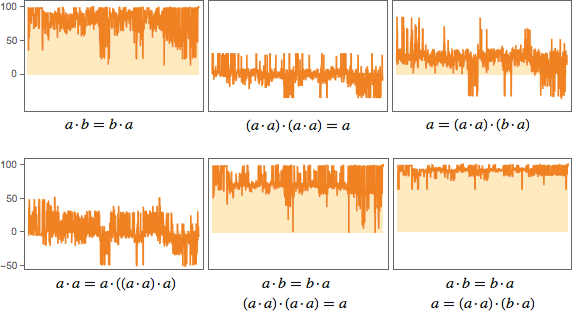a · b = b · a is very successful, often cutting down the proof by nearly 100 steps. (a · a) · (a · a) = a is much less successful; in fact, it actually sometimes seems to “confuse” FindEquationalProof, causing it to take more rather than fewer steps (visible as negative values in the plot). (a · a) · (b · a) = a is OK at shortening, but not as good as a · b = b · a. Though if one combines it with a · b = b · a, the result is more consistent shortening.

One could go on with this analysis, say including a comparison of how much shortening is produced by a given lemma, relative to how long its own proof was. But the problem is that if one adds several “useful lemmas”, like a · b = b · a and (a · a) · (b · a) = a, there are still plenty of long proofs—and thus a lot left to “understand”: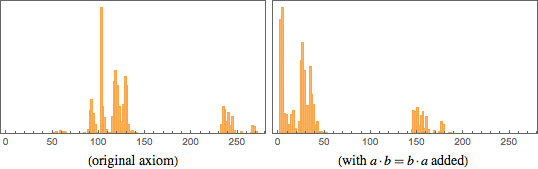## What Can One Understand?

There are different ways to create models of things. For a few hundred years, exact science was dominated by the idea of finding mathematical equations that could be solved to say how things should behave. But in pretty much the time since A New Kind of Science appeared, there’s been a strong shift to instead set up programs that can be run to say how things should behave.

Sometimes those programs are explicitly constructed for a particular purpose; sometimes they’re exhaustively searched for. And in modern times, at least one class of such programs is deduced using machine learning, essentially by going backwards from examples of how the system is known to behave.

OK, so with these different forms of modeling, how easy is it to “understand what’s going on”? With mathematical equations, it’s a big plus when it’s possible to find an “exact solution”—in which the behavior of the system can be represented by something like an explicit mathematical formula. And even when this doesn’t happen, it’s fairly common to be able to make at least some mathematical statements that are abstract enough to connect to other systems and other behaviors.

As I discussed above, with a program—like a cellular automaton—it can be a different story. Because it’s common to be thrust immediately into computational irreducibility, which ultimately limits how much one can ever hope to shortcut or “explain” what’s going on.

But what about with machine learning, and, say, with neural nets? At some level, the training of a neural net is like recapitulating inductive discovery in natural science. One’s trying to start from examples and deduce a model for how a system behaves. But then can one understand the model?

Again there are issues of computational irreducibility. But let’s talk about a case where we can at least imagine what it would look like to understand what’s going on.

Instead of using a neural net to model how some system behaves, let’s consider making a neural net that classifies some aspect of the world: say, takes images and classifies them according to what they’re images of (“boat”, “giraffe”, etc.). When we train the neural net, it’s learning to give correct final outputs. But potentially one can think of the way it does this as being to internally make a sequence of distinctions (a bit like playing a game of Twenty Questions) that eventually determines the correct output.

But what are those distinctions? Sometimes we can recognize some of them. “Is there a lot of blue in the image?”, for example. But most of the time they’re essentially features of the world that we humans don’t notice. Maybe there’s an alternative history of natural science where some of them would have shown up. But they’re not things that are part of our current canon of perception or analysis.

If we wanted to add them, we’d probably end up inventing words for them. But the situation is very similar to the one with the logic proof. An automated system has created things that it’s effectively using as “waypoints” in generating a result. But they’re not waypoints we recognize or relate to.

Once again, if we found that particular distinctions were very common for neural nets, we might decide that those are distinctions that are worth us humans learning, and adding to our standard canon of ways to describe the world.

Can we expect that a modest number of such distinctions would go a long way? It’s analogous to asking whether a modest number of theorems would go a long way in understanding something like the logic proof.

My guess is that the answer is fuzzy. If one looks, for example, at a large corpus of math papers, one can ask how common different theorems are. It turns out that the frequency of theorems follows an almost perfect Zipf law (with the Central Limit Theorem, the Implicit Function Theorem and Fubini’s Theorem as the top three). And it’s probably the same with distinctions that are “worth knowing”, or new theorems that are “worth knowing”.

Knowing a few will get one a certain distance, but there’ll be an infinite power-law tail, and one will never get to the end.

## The Future of Knowledge

Whether one looks at mathematics, science or technology, one sees the same basic qualitative progression of building a stack of increasing abstraction. It would be nice to be able to quantify this process. Perhaps one could look at how certain terms or descriptions that are common at one time later get subsumed into higher levels of abstraction, which then in turn have new terms or descriptions associated with them.

Maybe one could create an idealized model of this process using some formal model of computation, like Turing machines. Imagine that at the lowest level one has a basic Turing machine, with no abstraction. Now imagine selecting programs for this Turing machine according to some defined random process. Then run these programs and analyze them to see what “higher-level” model of computation can successfully reproduce the aggregate behavior of these programs without having to run each step in each program.

One might have thought that computational irreducibility would imply that this higher-level model of computation would inevitably be more complicated in its construction. But the key point is that we’re only trying to reproduce the aggregate behavior of the programs, not their individual behavior.

OK, but so then what happens if you iterate this process—essentially recapitulating idealized human intellectual history and building a progressive tower of abstraction?

Conceivably there’s some analogy to critical phenomena in physics, and to the renormalization group. And if so, one might imagine being able to identify a definite trajectory in the space of what amount to concept representation frameworks. What will the trajectory do?

Maybe it’ll have some kind of fixed-point behavior, representing the guess that at any point in history there are about the same number of abstract concepts that are worth learning—with new ones slowly being invented, and old ones being subsumed.

What might any of this mean for mathematics? One guess might be that any “random fact of mathematics”, say discovered empirically, would eventually be covered when some level of abstraction is reached. It’s not obvious how this process would work. After all, at any given level of abstraction, there are always new empirical facts to be “jumped to”. And it might very well be that the “rising tide of abstraction” would move only slowly compared to the rate at which such jumps could be made.

## The Future of Understanding

OK, so what does all this mean for the future of understanding?

In the past, when humans looked, say, at the natural world, they had few pretensions to understand it. Sometimes they would personify certain aspects of it in terms of spirits or deities. But they saw it as just acting as it did, without any possibility for humans to understand in detail why.

But with the rise of modern science—and especially as more of our everyday existence came to be in built environments dominated by technology (or regulatory structures) that we had designed—these expectations changed. And as we look at computation or AI today, it seems unsettling that we might not be able to understand it.

But ultimately there’s always going to be a competition between what the systems in our world do, and what our brains are capable of computing about them. If we choose to interact only with systems that are computationally much simpler than our brains, then, yes, we can expect to use our brains to systematically understand what the systems are doing.

But if we actually want to make full use of the computational capabilities that our universe makes possible, then it’s inevitable that the systems we’re dealing with will be equivalent in their computational capabilities to our brains. And this means that—as computational irreducibility implies—we’ll never be able to systematically “outthink” or “understand” those systems.

But then how can we use them? Well, pretty much like people have always used systems from the natural world. Yes, we don’t know everything about how they work or what they might do. But at some level of abstraction we know enough to be able to see how to get purposes we care about achieved with them.

What about in an area like mathematics? In mathematics we’re used to building our stack of knowledge so that each step is something we can understand. But experimental mathematics—as well as things like automated theorem proving—make it clear that there are places to go that won’t have this feature.

Will we call this “mathematics”? I think we should. But it’s a different tradition from what we’ve mostly used for the past millennium. It’s one where we can still build abstractions, and we can still construct new levels of understanding.

But somewhere underneath there will be all sorts of computational irreducibility that we’ll never really be able to bring into the realm of human understanding. And that’s basically what’s going on in the proof of my little axiom for logic. It’s an early example of what I think will be the dominant experience in mathematics—and a lot else—in the future.